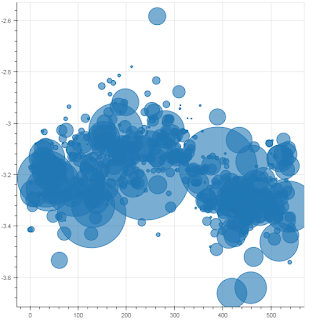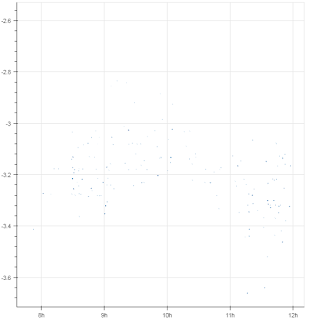# Scatter NOT Plotting Fill Radius Bubbles properly on datetime axis vs integer index axis

Hello Bokeh Users,

I searched for an answer to this question as I imagine someone else must have encountered it before. I have a pandas dataframe that I want to plot. I prefer to plot this data with “x_axis_type=‘datetime’”. However, the scatter radius bubbles aren’t plotted proportionally when I convert to datetime axis.

Here’s the plot with an integer indexed x-axis:Here’s the plot with the same data except for x-axis is converted to a datetime axis:You might be able to faintly notice the smallest specs of fill bubbles. They’re there but there small.

I tried to magnify the bubble size on the datetime index because I figured the datetime timestamp may be magnifying the proportions on the x-axis size. That didn’t work.

Thanks,

Pete

I forgot to add my code snippets and I’ll include those below… sorry about that…

Hello Bokeh Users,

I searched for an answer to this question as I imagine someone else must have encountered it before. I have a pandas dataframe that I want to plot. I prefer to plot this data with “x_axis_type=‘datetime’”. However, the scatter radius bubbles aren’t plotted proportionally when I convert to datetime axis.

Here’s the plot with an integer indexed x-axis:# esq variable is my list of radius sizes

x_data = range(0, len(est))

p = figure()

Here’s the plot with the same data except for x-axis is converted to a datetime axis:# esq variable is my list of radius sizes

p = figure(x_axis_type=“datetime”)

···

On Monday, July 3, 2017 at 1:15:46 PM UTC-5, Peter West wrote:

You might be able to faintly notice the smallest specs of fill bubbles. They’re there but there small.

I tried to magnify the bubble size on the datetime index because I figured the datetime timestamp may be magnifying the proportions on the x-axis size. That didn’t work.

Thanks,

Pete

Hi,

This is due to the fact that the “data” aspect ratio is very far from 1-1, see e.g.

``````[https://github.com/bokeh/bokeh/issues/517](https://github.com/bokeh/bokeh/issues/517)
``````

For some discussion. But basically boils down too: Circles are radially symmetric, but to actually draw them on the canvas, the radius has to be measured along some single dimension. By default, Bokeh uses the x-dimenson scale to map data distances to screen (pixel) distances for circles, which in your case results in the tiny circles (because the x-dimension is really “milliseconds since epoch” .

There are a couple of options:

Or, as an aside, consider a different approach to the visualization altogether. I would suggest in general that a bubble chart is not advised then whenever the scales of the x- and y- dimensions are not exactly identical.

Thanks,

Bryan

···

On Jul 3, 2017, at 13:15, Peter West [email protected] wrote:

Hello Bokeh Users,

I searched for an answer to this question as I imagine someone else must have encountered it before. I have a pandas dataframe that I want to plot. I prefer to plot this data with “x_axis_type=‘datetime’”. However, the scatter radius bubbles aren’t plotted proportionally when I convert to datetime axis.

Here’s the plot with an integer indexed x-axis:

Here’s the plot with the same data except for x-axis is converted to a datetime axis:

You might be able to faintly notice the smallest specs of fill bubbles. They’re there but there small.

I tried to magnify the bubble size on the datetime index because I figured the datetime timestamp may be magnifying the proportions on the x-axis size. That didn’t work.

Thanks,
Pete

You received this message because you are subscribed to the Google Groups “Bokeh Discussion - Public” group.
To unsubscribe from this group and stop receiving emails from it, send an email to [email protected].
To post to this group, send email to [email protected].
To view this discussion on the web visit https://groups.google.com/a/continuum.io/d/msgid/bokeh/3096735e-1317-4385-985c-52b2695b92f3%40continuum.io.

Thanks, Bryan.

I could use size and come up with some values in the following format:

dot_size = 4

ebq_max = np.max(eb.size)

ebt = list(eb.index)

ebp = list(eb.price)

ebq = list(eb.size / ebq_max * dot_size)

The ratio is locked to dot_size and the visualization stays the same even though I use different dot_size variables.

I may tinker around and come up with a better solution. I will post if I figure out a better way. Thanks for the great help thus far.

···

On Monday, July 3, 2017 at 1:15:46 PM UTC-5, Peter West wrote:

Hello Bokeh Users,

I searched for an answer to this question as I imagine someone else must have encountered it before. I have a pandas dataframe that I want to plot. I prefer to plot this data with “x_axis_type=‘datetime’”. However, the scatter radius bubbles aren’t plotted proportionally when I convert to datetime axis.

Here’s the plot with an integer indexed x-axis:Here’s the plot with the same data except for x-axis is converted to a datetime axis:You might be able to faintly notice the smallest specs of fill bubbles. They’re there but there small.

I tried to magnify the bubble size on the datetime index because I figured the datetime timestamp may be magnifying the proportions on the x-axis size. That didn’t work.